# GSEB Solutions Class 6 Maths Chapter 7 Fractions InText Questions

Gujarat Board GSEB Textbook Solutions Class 6 Maths Chapter 7 Fractions InText Questions and Answers.

## Gujarat Board Textbook Solutions Class 6 Maths Chapter 7 Fractions InText Questions

Try These (Page 137)

Question 1.
Show $$\frac { 3 }{ 5 }$$ on a number line.
Solution:
We divide the length between 0 and 1 on a number line into 5 equal parts. The point A represents $$\frac { 3 }{ 8 }$$Question 2.
Show $$\frac { 1 }{ 10 }$$ , $$\frac { 0 }{ 10 }$$ , $$\frac { 5 }{ 10 }$$ and $$\frac { 10 }{ 10 }$$ on a number line.
Solution:
We draw a number line. Divide the length between 0 and 1 into 10 equal parts.The point B represents $$\frac { 1 }{ 10 }$$
The point A represents $$\frac { 0 }{ 10 }$$
The point C represents $$\frac { 5 }{ 10 }$$
The point D represents $$\frac { 10 }{ 10 }$$Question 3.
Can you show any other fraction between 0 and 1? Write five more fractions that you can show and depict them on the number line.
Solution:
Yes, we can show any number of fractions that are greater than 0 and less than 1.
Five other fractions, i.e. $$\frac { 2 }{ 3 }$$ , $$\frac { 6 }{ 7 }$$ , $$\frac { 1 }{ 8 }$$ , $$\frac { 4 }{ 9 }$$ and $$\frac { 4 }{ 5 }$$ can be shown on a number line between 0 and 1. Direct these numbers on the number line by yourself.Question 4.
How many fractions lie between 0 and 1? Think, discuss and write your answer.
Solution:
An infinite number of fractions lie between 0 and 1.

Try These (Page 138)

Question 1.
Give a proper fraction:
(a) Whose numerator is 5 and denominator is 7.
(b) Whose denominator is 9 and numerator is 5.
(c) Whose numerator and denominator add up to 10. How many fractions of this kind can you make?
(d) Whose denominator is 4 more than the numerator.
(Give any five. How many more can you make?)
Solution:∴ Fractions whose numerator and denominator add up to 10 are: $$\frac { 0 }{ 10 }$$ , $$\frac { 1 }{ 9 }$$ , $$\frac { 2 }{ 8 }$$ , $$\frac { 3 }{ 7 }$$ and $$\frac { 4 }{ 6 }$$.
(d) There can be infinite number of fractions whose denominator is 4 more than the numerator. Some of them are: $$\frac { 1 }{ 5 }$$ , $$\frac { 2 }{ 6 }$$ , $$\frac { 3 }{ 7 }$$ , $$\frac { 4 }{ 8 }$$ , $$\frac { 5 }{ 9 }$$, etc.Question 2.
A fraction is given. How will you decide, by just looking at it, whether, the fraction is (a) less than 1? (b) equal to 1?
Solution:Question 3.
Fill up using one of these: ‘>’ , ‘<‘ or ‘=’Solution: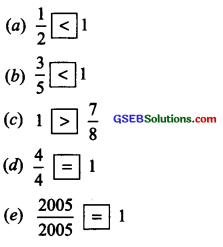Try These (Page 142)

Question 1.
Are $$\frac { 1 }{ 3 }$$ and $$\frac { 2 }{ 7 }$$;$$\frac { 2 }{ 5 }$$ and $$\frac { 2 }{ 7 }$$;$$\frac { 2 }{ 9 }$$ and $$\frac { 6 }{ 27 }$$ equivalent? Give reason.
Solution:
(i) $$\frac { 1 }{ 3 }$$ and $$\frac { 2 }{ 7 }$$ ∵ 1 × 7 = 7, 3 × 2 = 6
and 7 ≠ 6, i.e. 1 × 7 ≠ 3 × 2
∴ $$\frac { 1 }{ 3 }$$ and $$\frac { 2 }{ 7 }$$ are not equivalent fractions.

(ii) $$\frac { 2 }{ 5 }$$ and $$\frac { 2 }{ 7 }$$ ∵ 2 × 7 = 14, 5 × 2 = 10
and 14 ≠ 10, i.e. 2 × 7 ≠ 5 × 2
∴ $$\frac { 2 }{ 5 }$$ and $$\frac { 2 }{ 7 }$$ are not equivalent fractions.

(iii) $$\frac { 2 }{ 9 }$$ and $$\frac { 6 }{ 27 }$$ ∵ 2 × 27 = 54, 9 × 6 = 54
and 54 = 54, i.e. 2 × 27 = 9 × 6
∴ $$\frac { 2 }{ 9 }$$ and $$\frac { 6 }{ 27 }$$ are equivalent fractions.Question 2.
Give an example of four equivalent fractions.
Solution:
The following pairs of fractions are equivalent:
(i) $$\frac { 2 }{ 8 }$$ and $$\frac { 1 }{ 4 }$$
(ii) $$\frac { 5 }{ 12 }$$ and $$\frac { 15 }{ 36 }$$
(iii) $$\frac { 8 }{ 11 }$$ and $$\frac { 16 }{ 22 }$$
(iv) $$\frac { 4 }{ 26 }$$ and $$\frac { 2 }{ 13 }$$

Question 3.
Identify the fractions in each. Are these fractions equivalent?Solution:
(i) The given figure represents the fraction $$\frac { 6 }{ 8 }$$
We have: $$\frac { 6 }{ 8 }$$ = $$\frac{6 \div 2}{8 \div 2}$$ = $$\frac { 3 }{ 4 }$$

(ii) The given figure represents the fraction $$\frac { 9 }{ 12 }$$
We have: $$\frac { 9 }{ 12 }$$ = $$\frac{9 \div 3}{12 \div 3}$$ = $$\frac { 3 }{ 4 }$$

(iii) The given figure represents the fraction $$\frac { 12 }{ 16 }$$
We have: $$\frac { 12 }{ 16 }$$ = $$\frac{12 \div 4}{16 \div 4}$$ = $$\frac { 3 }{ 4 }$$

(iv) The given figure represents the fraction $$\frac { 15 }{ 20 }$$
We have: $$\frac { 12 }{ 16 }$$ = $$\frac{12 \div 4}{16 \div 4}$$ = $$\frac { 3 }{ 4 }$$
Since, all the fractions represent $$\frac { 3 }{ 4 }$$,
i.e., $$\frac { 6 }{ 8 }$$ = $$\frac { 9 }{ 12 }$$ = $$\frac { 12 }{ 16 }$$ = $$\frac { 15 }{ 20 }$$ =Thus, the given figures represent equivalent fractions.

Try These (Page 143)

Question 1.
Find five equivalent fractions of each of the following:
(i) $$\frac { 2 }{ 3 }$$
(ii) $$\frac { 1 }{ 5 }$$
(iii) $$\frac { 3 }{ 5 }$$
(iv) $$\frac { 5 }{ 9 }$$
Solution:Try These (Page 146)

Question 1.
Write the simplest form of:
(i) $$\frac { 15 }{ 75 }$$
(ii) $$\frac { 16 }{ 72 }$$
(iii) $$\frac { 17 }{ 51 }$$
(iv) $$\frac { 42 }{ 28 }$$
(v) $$\frac { 80 }{ 24 }$$
Solution:
(i) $$\frac { 15 }{ 75 }$$
Factors of 15 are: 1, 3, 5 and 15
Factors of 75 are: 1, 3, 5, 15, 25 and 75
Common factors are: 1, 3, 5 and 15
∴ HCF of 15 and 75 = 15
Now, $$\frac { 15 }{ 75 }$$ = $$\frac{15 \div 15}{75 \div 15}$$ = $$\frac { 1 }{ 5 }$$
Thus, simplest form of $$\frac { 15 }{ 75 }$$ is $$\frac { 1 }{ 5 }$$

(ii) $$\frac { 16 }{ 72 }$$
Factors of 16 are: 1, 2, 4, 6, 8 and 16
Factors of 72 are: 1, 2, 3, 4, 6, 8, 9, 12, 18, 24, 36 and 72
∴ Common factors are: 1, 2, 4, 6 and 8
∴ HCF of 16 and 72 is 8.
Now $$\frac { 16 }{ 72 }$$ = $$\frac{16 \div 8}{72 \div 8}$$ = $$\frac { 2 }{ 9 }$$
Thus, the simplest form of $$\frac { 16 }{ 72 }$$ is $$\frac { 2 }{ 9 }$$

(iii) $$\frac { 17 }{ 51 }$$
Factors of 17 are: 1 and 17
Factors of 51 are: 1, 3 and 17
The common factor is 17
∴ HCF of 17 and 51 = 17
Now $$\frac { 17 }{ 51 }$$ = $$\frac{17 \div 17}{51 \div 17}$$ = $$\frac { 1 }{ 3 }$$
Thus, the simplest form of $$\frac { 17 }{ 51 }$$ is $$\frac { 1 }{ 3 }$$

(iv) $$\frac { 42 }{ 28 }$$
Factors of 42 are: 1, 2, 3, 6, 7, 14, 21 and 42
Factors of 28 are: 1, 2, 4, 7, 14 and 28
Common factors are: 1, 2, 7 and 14
∴ HCF of 42 and 28 = 14
Now $$\frac { 42 }{ 28 }$$ = $$\frac{42 \div 14}{28 \div 14}$$ = $$\frac { 3 }{ 2 }$$
Thus, the simplest form of $$\frac { 42 }{ 28 }$$ is $$\frac { 3 }{ 2 }$$

(v) $$\frac { 80 }{ 24 }$$
Factors of 80 are: 1, 2, 4, 5, 8, 10, 16, 20, 40 and 80
Factors of 24 are: 1, 2, 3, 4, 6, 8, 12 and 24
Common factors are: 1, 2, 4 and 8
∴ HCF of 80 and 24 = 8
Now, $$\frac { 80 }{ 24 }$$ = $$\frac{80 \div 8}{24 \div 8}$$ = $$\frac { 10 }{ 3 }$$
Thus, the simplest form $$\frac { 80 }{ 24 }$$ is $$\frac { 10 }{ 3 }$$Question 2.
Is $$\frac { 49 }{ 64 }$$ in its simplest form?
Solution:
∵ Factors of 49 are: 1,7 and 49
Factors of 64 are: 1, 2, 4, 8, 16, 32 and 64
The common factor is 1, i.e. 49 and 64 have no common factor other than 1.
∴ 49 and 64 are coprime, i.e. $$\frac { 49 }{ 64 }$$ is in its simplest form.

Try These (Page 148)

Question 1.
You get one-fifth of a bottle of juice and your sister gets one-third of a bottle of juice. Who gets more?
Solution:
Let us divide a rectangle into 5 equal parts and shade one of them, i.e. one-fifth is shaded.Now, divide the same rectangle into 3 equal parts and shade one of them, Le. one-third is shaded, obviously, one third is greater. Thus, your sister gets more juice.

Try These (Page 149)

Question 1.
(a) Which is the larger fraction?
(i) $$\frac { 7 }{ 10 }$$ or $$\frac { 8 }{ 10 }$$
(ii) $$\frac { 11 }{ 24 }$$ or $$\frac { 13 }{ 24 }$$
(iii) $$\frac { 17 }{ 102 }$$ or $$\frac { 12 }{ 102 }$$

(b) Why are these comparisons easy to make?
Solution:
(a) (i) $$\frac { 7 }{ 10 }$$ < $$\frac { 8 }{ 10 }$$
Denominators are same and 7 < 8
∴ $$\frac { 7 }{ 10 }$$ < $$\frac { 8 }{ 10 }$$

(ii) $$\frac { 11 }{ 24 }$$ and $$\frac { 13 }{ 24 }$$
Denominators are same and 11 < 13
∴ $$\frac { 11 }{ 24 }$$ < $$\frac { 13 }{ 24 }$$

(iii) $$\frac { 17 }{ 102 }$$ and $$\frac { 12 }{ 102 }$$
Denominators are same and 17 > 12
∴ $$\frac { 17 }{ 102 }$$ and $$\frac { 12 }{ 102 }$$
(b) These comparisons are easy because these are like fractions.Question 2.
(a) $$\frac { 1 }{ 8 }$$ , $$\frac { 5 }{ 8 }$$ , $$\frac { 3 }{ 8 }$$
(b) $$\frac { 1 }{ 5 }$$ , $$\frac { 11 }{ 5 }$$ , $$\frac { 4 }{ 5 }$$ , $$\frac { 3 }{ 5 }$$ , $$\frac { 7 }{ 5 }$$
(c) $$\frac { 1 }{ 7 }$$ , $$\frac { 3 }{ 7 }$$ , $$\frac { 13 }{ 7 }$$ , $$\frac { 11 }{ 7 }$$ , $$\frac { 7 }{ 7 }$$
Solution:
We can write the like fractions in ascending or in descending order according to the order of their numerators.
(a) $$\frac { 1 }{ 8 }$$ , $$\frac { 5 }{ 8 }$$ , $$\frac { 3 }{ 8 }$$
∵ 1 , 3 and 5 are in ascending order.
∴ $$\frac { 1 }{ 8 }$$ , $$\frac { 3 }{ 8 }$$ and $$\frac { 5 }{ 8 }$$ are in ascending order and $$\frac { 5 }{ 8 }$$ , $$\frac { 3 }{ 8 }$$ and $$\frac { 1 }{ 8 }$$ are in descending order.

(b) $$\frac { 1 }{ 5 }$$ , $$\frac { 11 }{ 5 }$$ , $$\frac { 4 }{ 5 }$$ , $$\frac { 3 }{ 5 }$$ , $$\frac { 7 }{ 5 }$$
∵ 1, 3, 4, 7 and 11 are in ascending order and 11, 7, 4, 3 and 1 are in descending order.
∴ Fractions in ascending order.
$$\frac { 1 }{ 7 }$$ , $$\frac { 3 }{ 7 }$$ , $$\frac { 7 }{ 7 }$$ , $$\frac { 11 }{ 7 }$$ , $$\frac { 13 }{ 7 }$$

Try These (Page 151)

Question 1.
Arrange the following in ascending and descending order:
(a) $$\frac { 1 }{ 12 }$$ , $$\frac { 1 }{ 23 }$$ , $$\frac { 1 }{ 5 }$$ , $$\frac { 1 }{ 7 }$$ , $$\frac { 1 }{ 50 }$$ , $$\frac { 1 }{ 9 }$$ , $$\frac { 1 }{ 17 }$$

(b) $$\frac { 3 }{ 7 }$$ , $$\frac { 3 }{ 11 }$$ , $$\frac { 3 }{ 5 }$$ , $$\frac { 3 }{ 2 }$$ , $$\frac { 3 }{ 13 }$$ , $$\frac { 3 }{ 4 }$$ , $$\frac { 3 }{ 17 }$$

(c) Write 3 more similar examples and arrange them in ascending and descending order.
Solution:
We know that in ‘unlike’ fractions having, same numerator, the greater the value of the denominator, the smaller the value of the fractional number.
(a) ∵ 50, 23, 17, 12, 9, 7 and 5 are in descending order
∴ Fractions in ascending order are:
$$\frac { 1 }{ 50 }$$ , $$\frac { 1 }{ 23 }$$ , $$\frac { 1 }{ 17 }$$ , $$\frac { 1 }{ 12 }$$ , $$\frac { 1 }{ 9 }$$ , $$\frac { 1 }{ 7 }$$ , $$\frac { 1 }{ 5 }$$

(b) ∵ 17, 13, 11, 7, 5, 4 and 2 are in descending order
∴ Ascending order of the given fractions is
$$\frac { 3 }{ 17 }$$ , $$\frac { 3 }{ 13 }$$ , $$\frac { 3 }{ 11 }$$ , $$\frac { 3 }{ 7 }$$ , $$\frac { 3 }{ 5 }$$ , $$\frac { 3 }{ 4 }$$ , $$\frac { 3 }{ 2 }$$
And descending order of the given fractions is
$$\frac { 3 }{ 2 }$$ , $$\frac { 3 }{ 4 }$$ , $$\frac { 3 }{ 5 }$$ , $$\frac { 3 }{ 7 }$$ , $$\frac { 3 }{ 11 }$$ , $$\frac { 3 }{ 13 }$$ , $$\frac { 3 }{ 17 }$$

(c) Three more examples of unlike fractions with the same numerator are:
(i) $$\frac { 2 }{ 13 }$$ , $$\frac { 2 }{ 25 }$$ , $$\frac { 2 }{ 6 }$$ , $$\frac { 2 }{ 8 }$$ , $$\frac { 2 }{ 10 }$$ , $$\frac { 2 }{ 17 }$$
(ii) $$\frac { 5 }{ 6 }$$ , $$\frac { 5 }{ 17 }$$ , $$\frac { 5 }{ 14 }$$ , $$\frac { 5 }{ 7 }$$ , $$\frac { 5 }{ 12 }$$ , $$\frac { 5 }{ 8 }$$ , $$\frac { 5 }{ 11 }$$
(iii) $$\frac { 4 }{ 11 }$$ , $$\frac { 4 }{ 13 }$$ , $$\frac { 4 }{ 5 }$$ , $$\frac { 4 }{ 7 }$$ , $$\frac { 4 }{ 19 }$$ , $$\frac { 4 }{ 17 }$$ , $$\frac { 4 }{ 15 }$$
Arrange the above in ascending and descending order by yourself
Solution:
(i) Ascending order:
$$\frac { 2 }{ 25 }$$ , $$\frac { 2 }{ 17 }$$ , $$\frac { 2 }{ 13 }$$ , $$\frac { 2 }{ 10 }$$ , $$\frac { 2 }{ 8 }$$ , $$\frac { 2 }{ 6 }$$
Descending order:
$$\frac { 2 }{ 6 }$$ , $$\frac { 2 }{ 8 }$$ , $$\frac { 2 }{ 10 }$$ , $$\frac { 2 }{ 13 }$$ , $$\frac { 2 }{ 17 }$$ , $$\frac { 2 }{ 25 }$$

(ii) Ascending order:
$$\frac { 5 }{ 17 }$$ , $$\frac { 5 }{ 14 }$$ , $$\frac { 5 }{ 12 }$$ , $$\frac { 5 }{ 11 }$$ , $$\frac { 5 }{ 8 }$$ , $$\frac { 5 }{ 7 }$$ , $$\frac { 5 }{ 6 }$$
Descending order:
$$\frac { 5 }{ 6 }$$ , $$\frac { 5 }{ 7 }$$ , $$\frac { 5 }{ 8 }$$ , $$\frac { 5 }{ 11 }$$ , $$\frac { 5 }{ 12 }$$ , $$\frac { 5 }{ 14 }$$ , $$\frac { 5 }{ 17 }$$

(iii) Ascending order:
$$\frac { 4 }{ 19 }$$ , $$\frac { 4 }{ 17 }$$ , $$\frac { 4 }{ 15 }$$ , $$\frac { 4 }{ 13 }$$ , $$\frac { 4 }{ 11 }$$ , $$\frac { 4 }{ 7 }$$ , $$\frac { 4 }{ 5 }$$
Descending order:
$$\frac { 4 }{ 5 }$$ , $$\frac { 4 }{ 7 }$$ , $$\frac { 4 }{ 11 }$$ , $$\frac { 4 }{ 13 }$$ , $$\frac { 4 }{ 15 }$$ , $$\frac { 4 }{ 17 }$$ , $$\frac { 4 }{ 19 }$$

Try These (Page 155)

Question 1.
My mother divided an apple into 4 equal parts. She gave me two parts and my brother one part. How many apples did she give to both of us together?
Solution:
On dividing an apple into 4 equal parts, each part of the apple will be 1/4. My mother gave me two parts, i.e., 2/4 parts, and my brother one part, i.e., 1/4 part. The part she gave us together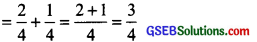Question 2.
Mother asked Neelu and her brother to pick stones from the wheat. Neelu picked one-fourth of the total stones in it and her brother also picked up one-fourth of the stones. What fraction of the stones did both pick up together?
Solution:
Neelu picked one-fourth of the total stones, i.e., 1/4 stones from the wheat and her brother picked up one-fourth of stones, i.e., 1/4 stones. Fractions of stones picked up together by them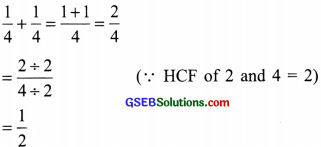Question 3.
Sohan was putting covers on his notebooks. He put one-fourth of the covers on Monday. He put another one-fourth on Tuesday and the remaining on Wednesday. What fraction of the covers did he put on Wednesday?
Solution:
Sohan put one-fourth of the covers on Monday, i.e., 1/4 covers. He put one-fourth on Tuesday, i.e., 1/4 covers and the remaining on Wednesday. Now, covers put on Monday and TuesdaySo, covers put on Wednesday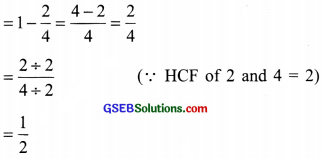Try These (Page 156)

Question 1.
Add with the help of a diagram.
(i) $$\frac { 1 }{ 8 }$$ + $$\frac { 1 }{ 8 }$$
(ii) $$\frac { 2 }{ 5 }$$ + $$\frac { 3 }{ 5 }$$
(iii) $$\frac { 1 }{ 6 }$$ + $$\frac { 1 }{ 6 }$$ + $$\frac { 1 }{ 6 }$$
Method I:
Look at the figure. It is divided into 8 equal parts.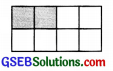Its shaded part = $$\frac { 2 }{ 8 }$$
i.e., $$\frac { 1 }{ 8 }$$ + $$\frac { 1 }{ 8 }$$ = $$\frac{1+1}{8}$$ = $$\frac { 2 }{ 8 }$$ or $$\frac { 1 }{ 4 }$$

Method II:
We can also represent the above sum in the following manner:∴ $$\frac { 1 }{ 8 }$$ + $$\frac { 1 }{ 8 }$$ = $$\frac{1+1}{8}$$ = $$\frac { 2 }{ 8 }$$

(ii) $$\frac { 2 }{ 5 }$$ + $$\frac { 3 }{ 5 }$$
We have: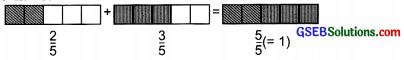Thus, $$\frac { 2 }{ 5 }$$ + $$\frac { 3 }{ 5 }$$ = $$\frac{2+3}{5}$$ = $$\frac { 5 }{ 5 }$$ = 1

(iii) $$\frac { 1 }{ 6 }$$ + $$\frac { 1 }{ 6 }$$ + $$\frac { 1 }{ 6 }$$
We have: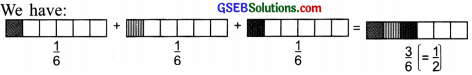Thus, $$\frac { 1 }{ 6 }$$ + $$\frac { 1 }{ 6 }$$ + $$\frac { 1 }{ 6 }$$ = $$\frac{1+1+1}{6}$$ = $$\frac { 3 }{ 6 }$$ = $$\frac { 1 }{ 2 }$$

Question 2.
add $$\frac { 1 }{ 12 }$$ + $$\frac { 1 }{ 12 }$$ How will we show this pictorially and by using paper folding?
Solution:
$$\frac { 1 }{ 12 }$$ + $$\frac { 1 }{ 12 }$$
We have:
$$\frac { 1 }{ 12 }$$ + $$\frac { 1 }{ 12 }$$ = $$\frac { 1+1 }{ 12 }$$ + $$\frac { 2 }{ 12 }$$ = $$\frac { 1 }{ 16 }$$
To show $$\frac { 1 }{ 12 }$$ + $$\frac { 1 }{ 12 }$$ pictorially, we have:Using paper folding (the activity): Do it yourself.Question 3.
Make 5 more examples of problems given in 1 and 2 above. Solve them with your friends.
Solution:
Do it yourself.

Try These (Page 157)

Question 1.
Find the difference between $$\frac { 7 }{ 8 }$$ and $$\frac { 3 }{ 8 }$$
Solution:
∵ $$\frac { 7 }{ 8 }$$ – $$\frac { 3 }{ 8 }$$ = $$\frac { 7-3 }{ 8 }$$ and $$\frac { 4 }{ 8 }$$ = $$\frac { 1 }{ 2 }$$
∴ The difference between $$\frac { 7 }{ 8 }$$ and $$\frac { 3 }{ 8 }$$ is $$\frac { 1 }{ 2 }$$.Question 2.
Mother made a good Patti in a round shape. She divided it into 5 parts. Seema ate one piece from it. If I eat another piece then how much would be left?
Solution:
Total number of equal parts of gud patti = 5
Eaten by Seema = $$\frac { 1 }{ 5 }$$
Eaten by me = $$\frac { 1 }{ 5 }$$
Fraction of gud patti eaten by Seema and me
= $$\frac { 1 }{ 5 }$$ + $$\frac { 1 }{ 5 }$$ = $$\frac { 1+1 }{ 5 }$$ = $$\frac { 2 }{ 5 }$$
∴ Fraction of gud patti left over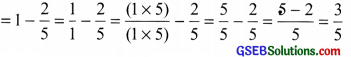Question 3.
My elder sister divided the watermelon into 16 parts. I ate 7 out of them. My friend ate 4. How much did we eat altogether? How much more of the watermelon did I eat than my friend? What portion of the watermelon remained?
Solution:
Total number of parts =16
The fraction of watermelon eaten∴ $$\frac { 3 }{ 16 }$$ parts are eaten by us.
Since,[Fraction of watermelon I ate] – [ Fraction of watermelon my friend ate]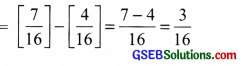∴ I ate $$\frac { 3 }{ 16 }$$ portion of watermelon more than my friend.
Now,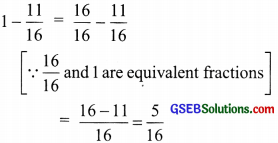Question 4.
Make five problems of this type and solve them with your friends.
Solution:
Do it yourself.

Try These (page 159)

Question 1.
Add $$\frac { 2 }{ 5 }$$ + $$\frac { 3 }{ 7 }$$
Solution:
$$\frac { 2 }{ 5 }$$ + $$\frac { 3 }{ 7 }$$
∵ LCM of 5 and 7 = 35Question 2.
Subtract $$\frac { 2 }{ 5 }$$ from $$\frac { 5 }{ 7 }$$
Solution:
$$\frac { 5 }{ 7 }$$ – $$\frac { 2 }{ 5 }$$
∵ LCM of 7 and 5 = 35